2018-10-24

## Statistical tests - continuous variables

• t-test:
• One-sample t-test
• (e.g. $$H_0$$: mean=5)
• Independent two-sample t-test
• (e.g. $$H_0$$: mean of sample 1 = mean of sample 2)
• Paired two-sample t-test
• (e.g. $$H_0$$: mean difference between pairs = 0)

## One-sample t-test: does mean = X?

• e.g. Question: Published data suggests that the failure rate for a particular piece of equipment from a supplier is 2.1%

• A research facility want to know if this holds true in their own lab?

## One-sample t-test: does mean = X?

• Null hypothesis, $$H_0$$:
• Mean monthly failure rate = 2.1%
• Alternative hypothesis: $$H_1$$:
• Mean monthly failure rate $$\ne$$ 2.1%
• Tails: two-tailed

• Either reject or do not reject the null hypothesis -

## One sample t-test; the data

       Month Monthly.failure.rate
1    January                 2.90
2   February                 2.99
3      March                 2.48
4      April                 1.48
5        May                 2.71
6       June                 4.17
7       July                 3.74
8     August                 3.04
9  September                 1.23
10   October                 2.72
11  November                 3.23
12  December                 3.40

## One-sample t-test; key assumptions

• Observations are independent
• Observations are normally distributed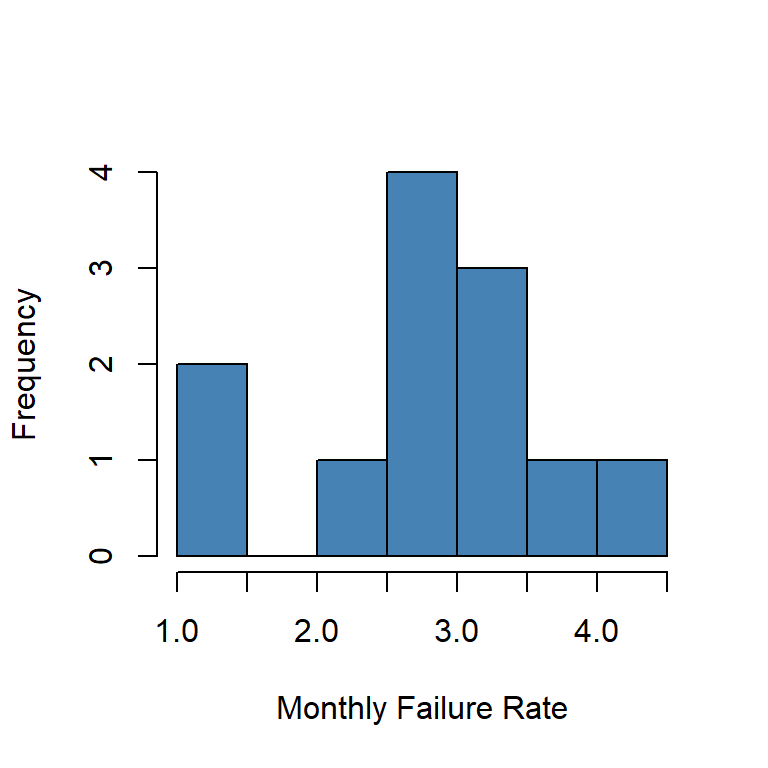## One sample t-test; the summary statistics

mean = $$(2.9 + \dots + 3.40) / 12$$ = 2.841

Standard deviation = 0.837

Hypothesised Mean = 2.1

## One-sample t-test; results

Test statistic: $t_{n-1} = t_{11} = \frac{\bar{x} - \mu_0} {s.d. / \sqrt{n}} = \frac{2.84 - 2.10}{s.e.(\bar{x})} =$3.065

## T-distributions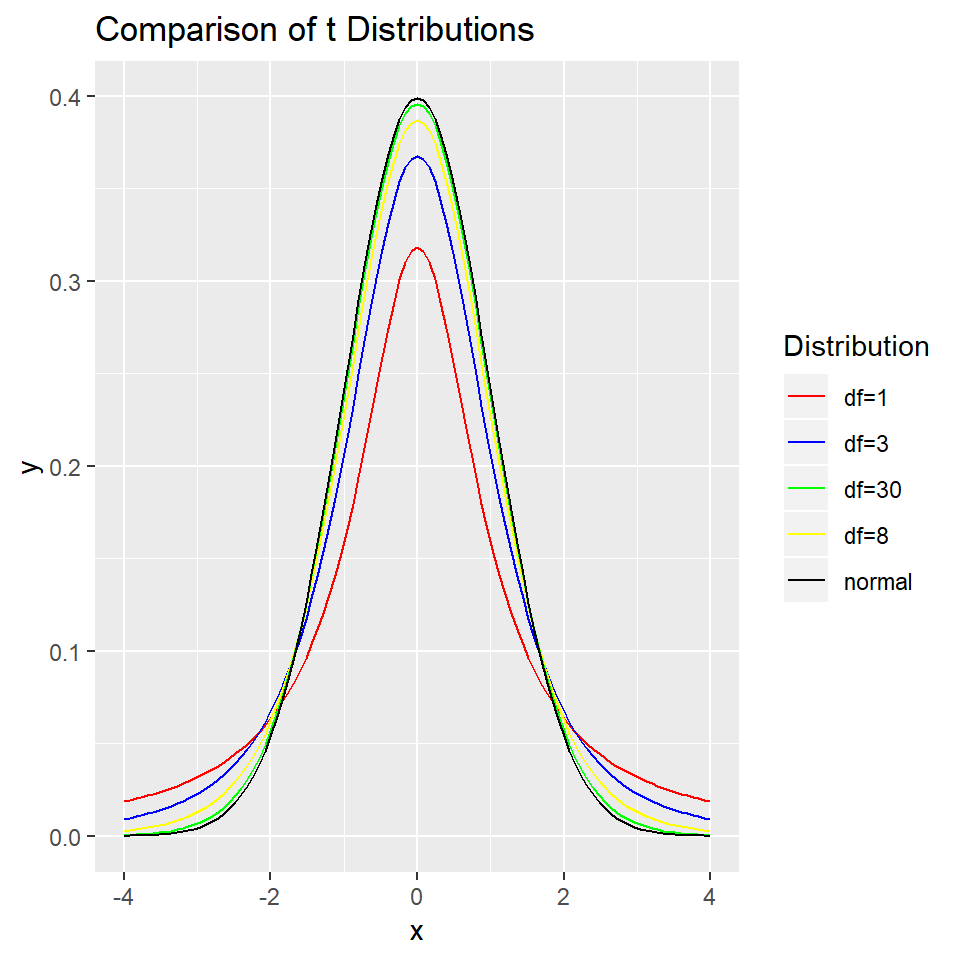## One-sample t-test; results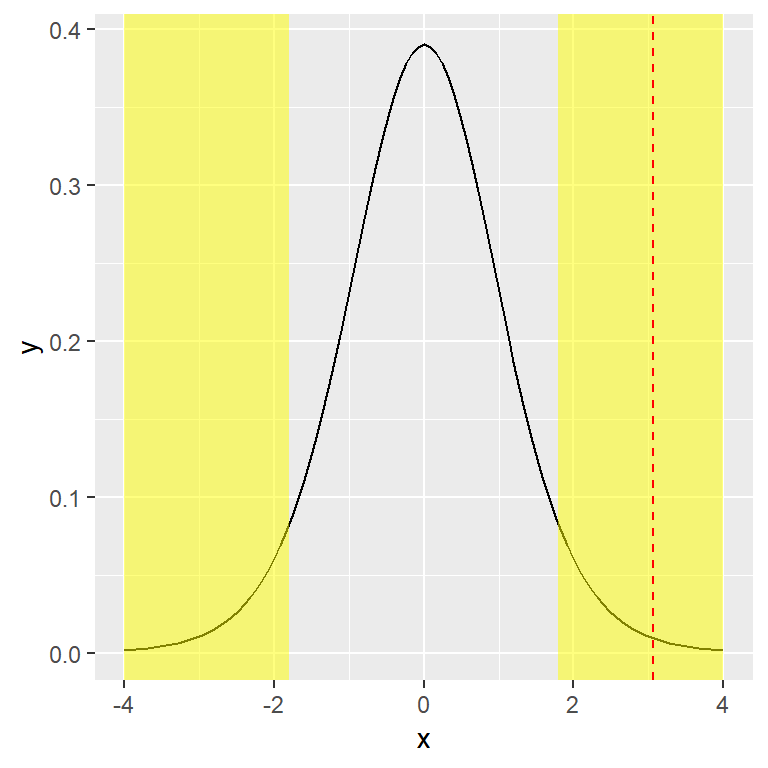## One-sample t-test; results

Test statistic: $t_{n-1} = t_{11} = \frac{\bar{x} - \mu_0} {s.d. / \sqrt{n}} = \frac{2.84 - 2.10}{s.e.(\bar{x})} =$3.065

df = 11 P = 0.01

Reject $$H_0$$ - Evidence that mean monthly failure rate $$\ne$$ 2.1%

## One-sample t-test; results

• The mean monthly failure rate of the equipement in the lab is 2.84
• It is not equal to the hypothesized mean proposed by the company of 2.1.
• t=3.07, df=11, p=0.01

## Two-sample t-test

• Two types of two-sample t-test:
• Independent:
• e.g.the weight of two different breeds of mice
• Paired
• e.g. a measurement of disease at two different parts of the body in the same patient / animal
• e.g. measurements before and after treatment for the same individual

## Independent two-sample t-test: Does the mean of group A = mean of group B?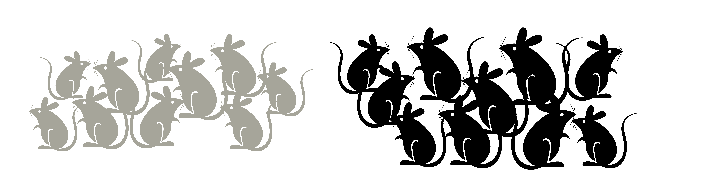• e.g. research question: 40 male mice (20 of breed A and 20 of breed B) were weighed at 4 weeks old

• Does the weight of 4-week old male mice depend on breed?

## Independent two-sample t-test: Does the mean of group A = mean of group B?

• Null hypothesis, $$H_0$$
• mean weight of breed A = mean weight of breed B
• Alternative hypothesis, $$H_1$$
• mean weight of breed B $$\ne$$ mean weight of breed B
• Tails: two-tailed
• Either reject or do not reject the null hypothesis -

## Independent two-sample t-test: the data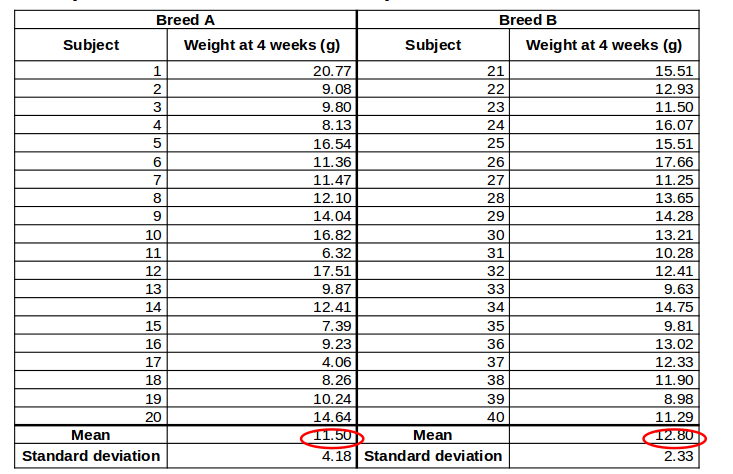## Independent two-sample t-test: key assumptions

• Observations are independent
• Observations are normally-distributed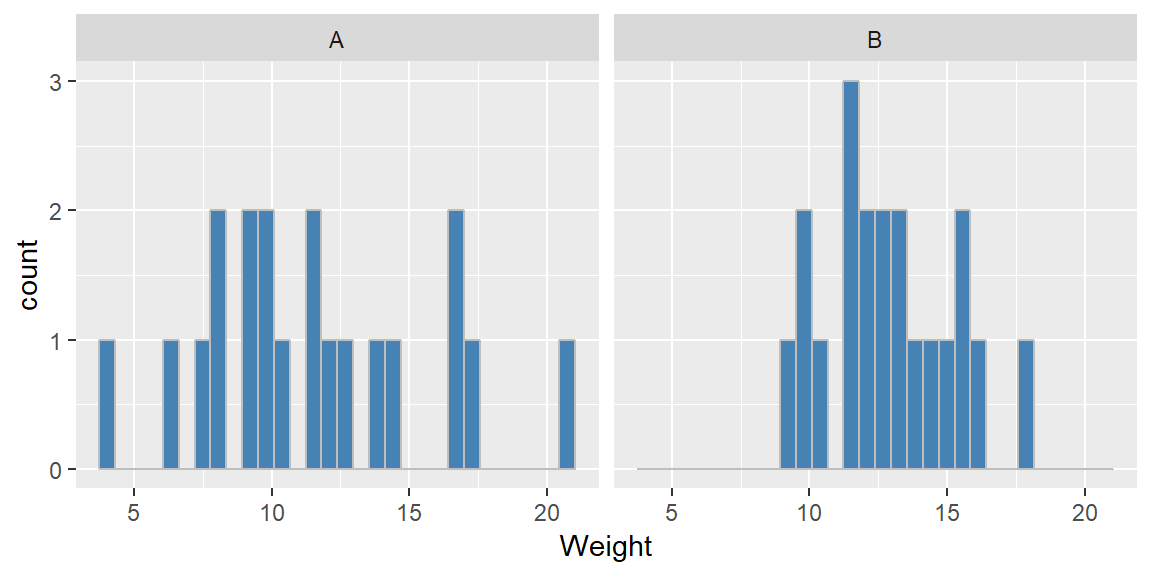## Independent two-sample t-test: More key assumptions

• Equal variance in the two comparison groups
• Use "Welch's correction" if variances are different
• alters the t-statistic and degrees of freedom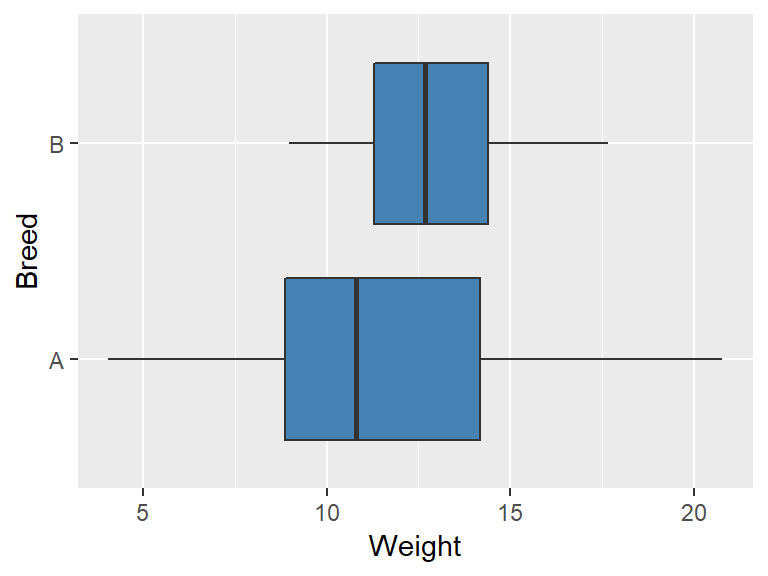## Independent two-sample t-test: result

$$t_{df} = \frac{\bar{X_A} - \bar{X_B}}{s.e.(\bar{X_A} - \bar{X_B})}$$ = -1.21

df = 29.78 (with Welch's correction)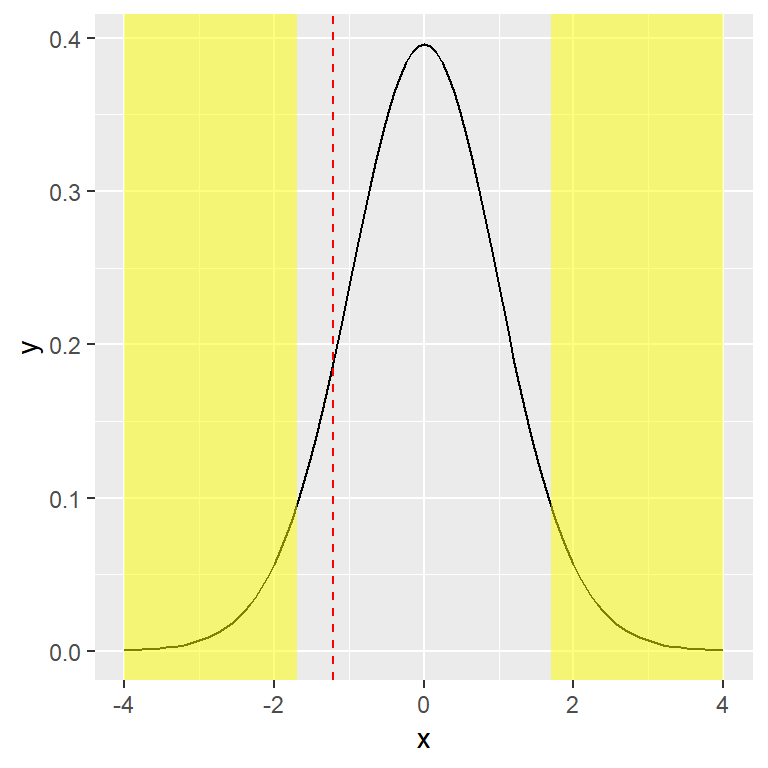P-value: 0.24

Do not reject $$H_0$$

(No evidence that mean weight of breed A $$\ne$$ mean weight of breed B)

## Independent two-sample t-test: result

• The difference in mean weight between the two breeds is -1.30
• [NB as this is negative, breed B mice tend to be bigger than breed A].
• There is no evidence of a difference in weights between breed A and breed B.
• t=-1.21, df= 29.78 (Welch’s correction), p=0.24

## Paired two-sample t-test: Does the mean difference = 0?

• e.g. Research question: 20 patients with ovarian cancer were studied using MRI imaging. Cellularity was measured for each patient at two sites of disease.
• Does the cellularity differ between two different sites of disease?
• cellularity is amount of tumour (versus normal cells)
• high cellularity means lots of tumour

## Paired two-sample t-test: Does the mean difference = 0?

• Null hypothesis, $$H_0$$:
• Cellularity at site A = Cellularity at site B
• Alternative hypothesis, $$H_1$$
• Cellularity at site A $$\ne$$ Cellularity at site B
• Tails: two-tailed
• Either reject or do not reject the null hypothesis

## Paired two-sample t-test; null hypothesis

• $$H_0$$; Cellularity at site A = Cellularity at site B
• or
• $$H_0$$: Cellularity at site A - Cellularity at site B = 0

## Paired two-sample t-test; the data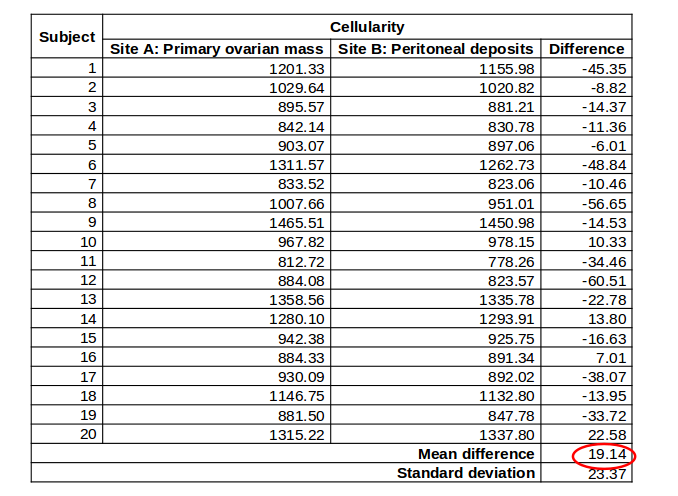## Paired two-sample t-test; key assumptions

• Observations are independent
• The paired differences are normally-distributed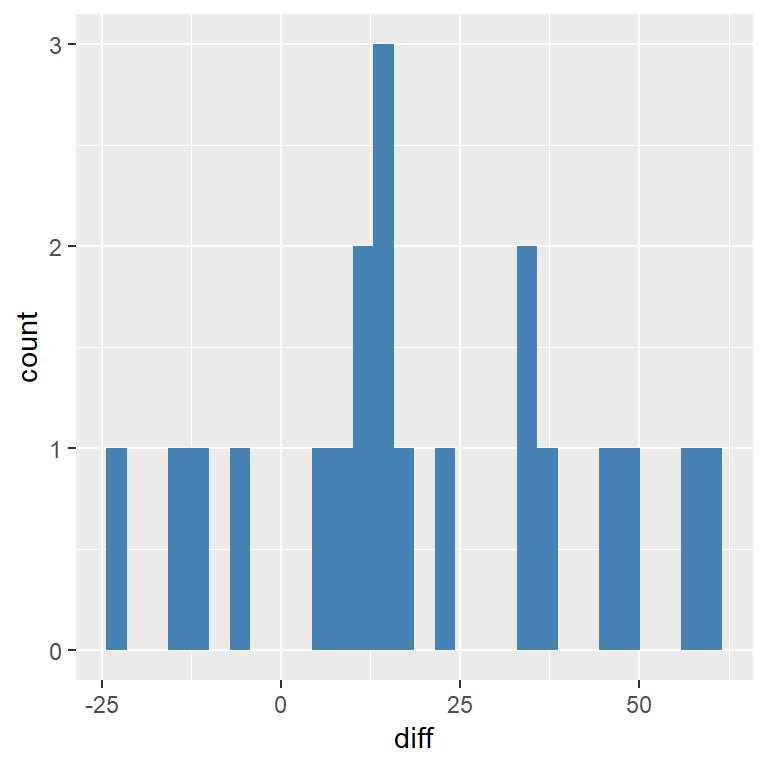## Paired two-sample t-test; results

$$t_{n-1} = t_{19} = \frac{\bar{X_{A-B}}}{s.e.(\bar{X_{A-B}})} =$$ 3.66

df = 19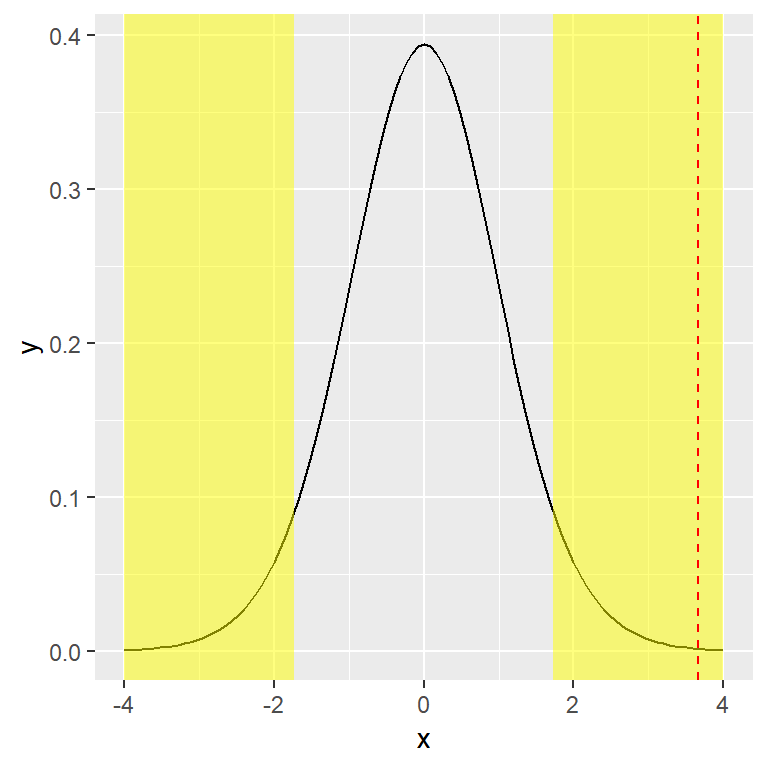P-value: 0.002

Reject $$H_0$$ (evidence that cellularity at Site A $$\ne$$ site B)

## Paired two-sample t-test; results

• The difference in cellularity between the two sites is 19.14 (95% CI: 8.20, 30.08).
• There is evidence of a difference in cellularity between the two sites.
• t=3.66, df=19, p=0.002.

## Extensions

• What if normality is not reasonable?
• Transform your data, e.g. log transformation
• Non-parametric tests….
• What if you have more than two groups?
• Approaches such as ANOVA
• What if you want to look at the relationship between two continuous variables
• Linear regression

## Summary - continuous variables

• One-sample t-test
• Use when we have one group.
• Independent two-sample t-test
• Use when we have two independent groups. A Welch correction may be needed if the two groups have different spread.
• Paired two-sample t-test
• Use when we have two non-independent groups.
• Non-parametric tests or transformations
• Use when we cannot assume normality.

## Summary - t-test

• Turn scientific question to null and alternative hypothesis